# Fidget Spinner RPM Counter

Arduino Project: How to make a Fidget Spinner RPM counter with Hall-effect sensor.

EasyProtip1 hour42,702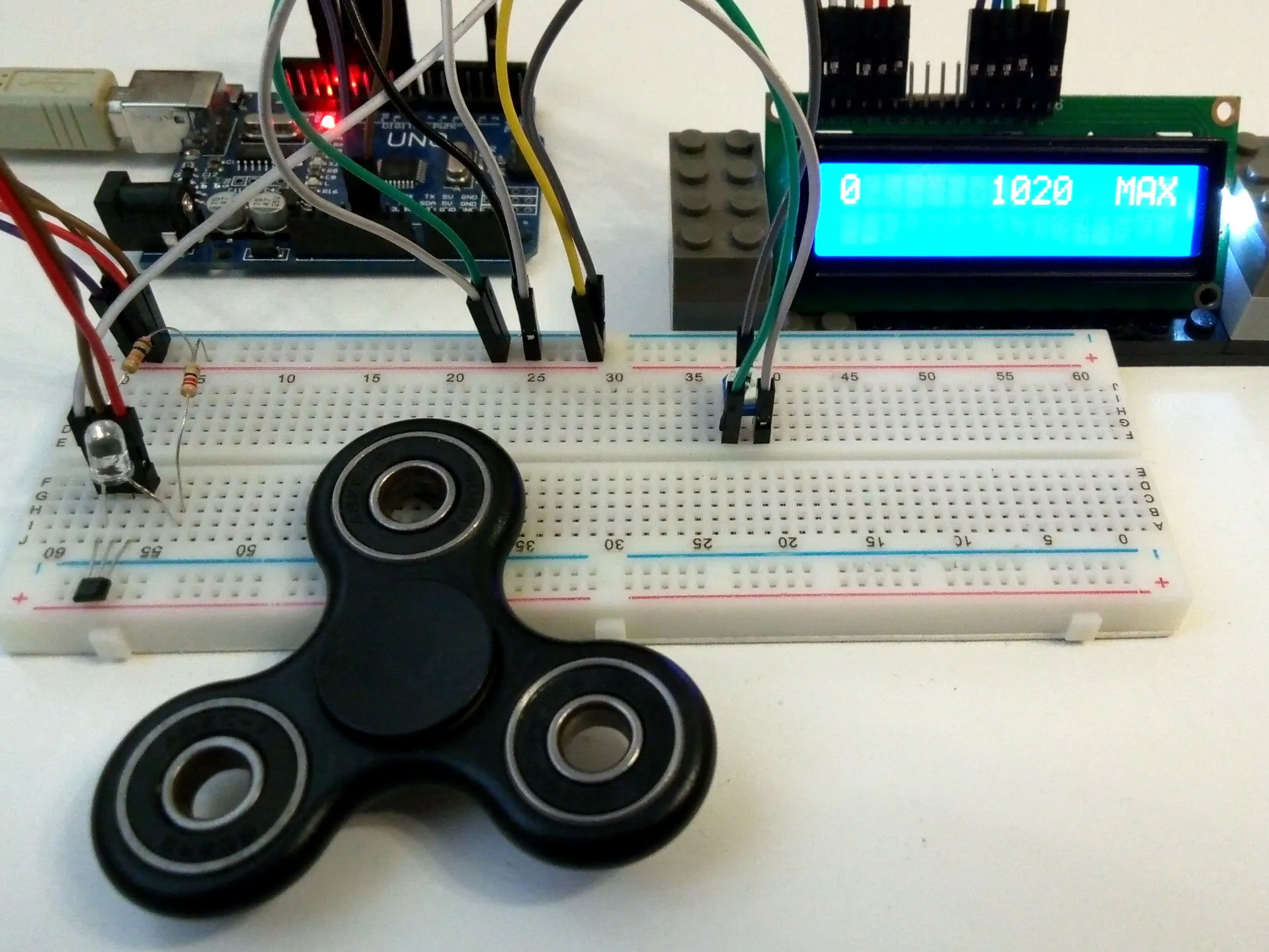## Things used in this project

### Hardware componentsArduino UNO & Genuino UNO
×1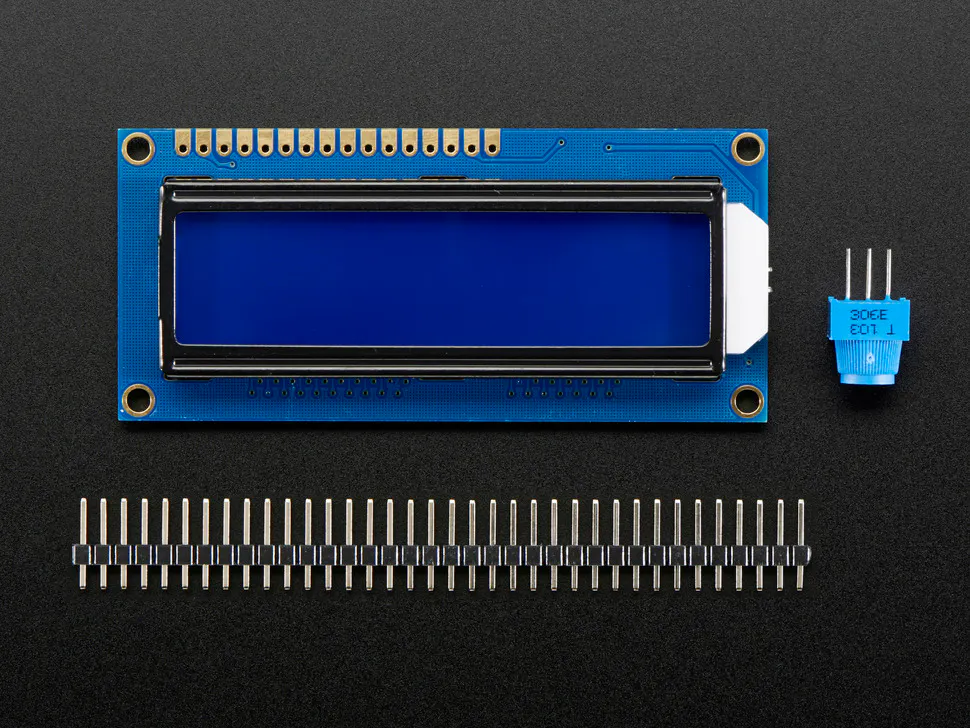Adafruit Standard LCD - 16x2 White on Blue
×1Resistor 10k ohm
×1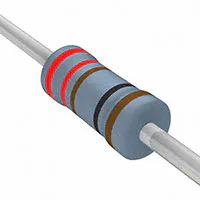Resistor 221 ohm
×1LED (generic)
×1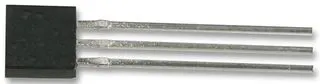Hall Effect Sensor
×1Jumper wires (generic)
×1

### Software apps and online servicesArduino IDE

## Schematics

### spinnerrpm_bb_7MRrxg3dQ0.jpg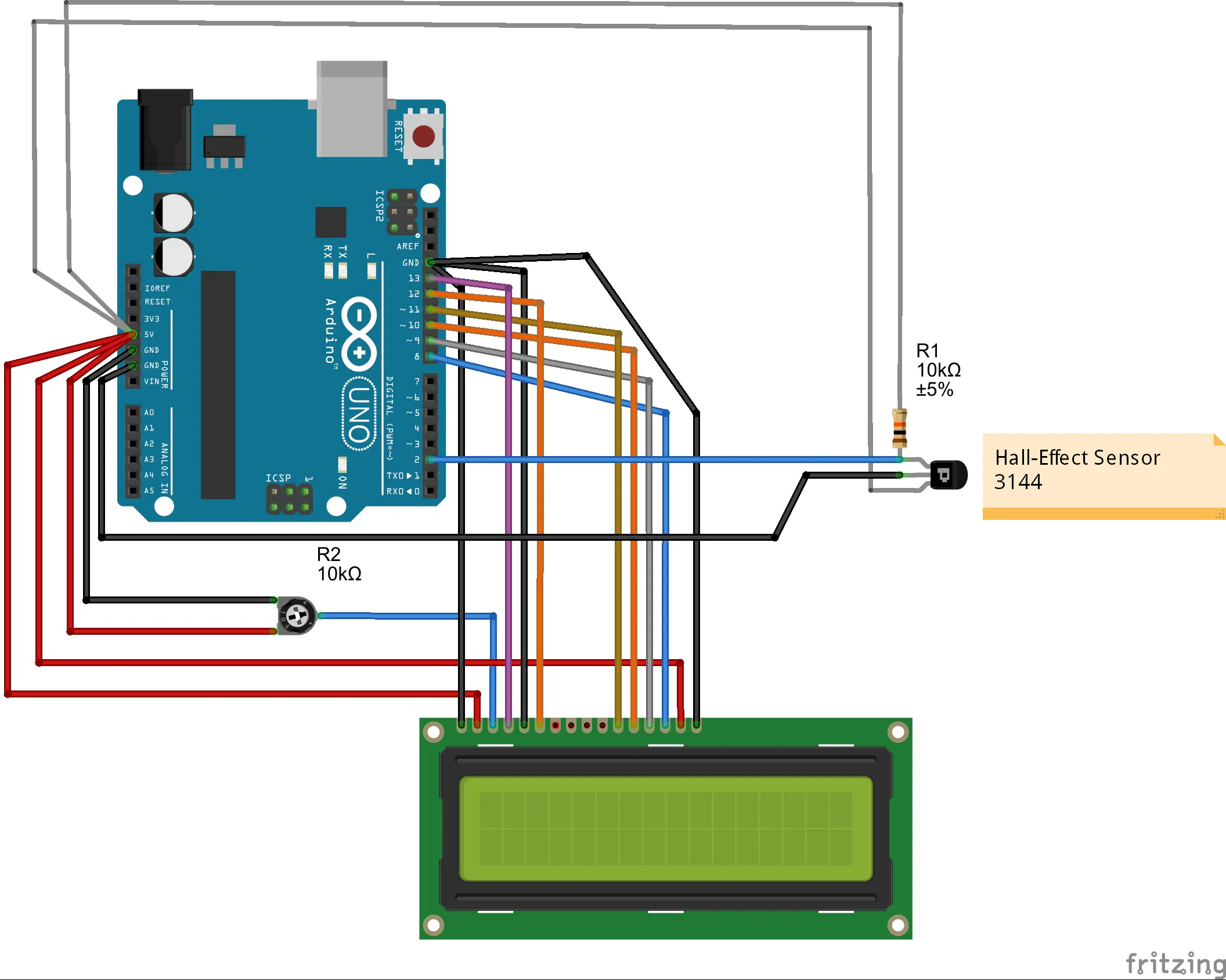## Code

### spinnerRPM_v2.2.ino

Arduino
```#include <LiquidCrystal.h>
LiquidCrystal lcd(13,12,11,10,9,8);
const int hallSensorPin = 2;                      // connect the hall effect sensor on pin 2
const unsigned long sampleTime = 1000;
const int maxRPM = 1260;                  // maximum RPM for LCD Bar
int rpmMaximum = 0;

void setup()
{
pinMode(hallSensorPin,INPUT);
Serial.begin(9600);
lcd.begin(16, 2);
lcd.print("Initializing");
delay(1000);
lcd.clear();
}

void loop()
{
delay(100);
int rpm = getRPM();
if (rpm > rpmMaximum) rpmMaximum = rpm;
lcd.clear();
displayRPM(rpm);
displayBar(rpm);

}

int getRPM()
{
int count = 0;
boolean countFlag = LOW;
unsigned long currentTime = 0;
unsigned long startTime = millis();
while (currentTime <= sampleTime)
{
if (digitalRead(hallSensorPin) == HIGH)
{
countFlag = HIGH;
}
if (digitalRead(hallSensorPin) == LOW && countFlag == HIGH)
{
count++;
countFlag=LOW;
}
currentTime = millis() - startTime;
}
int countRpm = int(60000/float(sampleTime))*count;
return countRpm;
}

void displayRPM(int rpm)
{
lcd.clear();
lcd.setCursor(0, 0);
lcd.print(rpm,DEC);
lcd.setCursor(7,0);
lcd.print(rpmMaximum, DEC);
lcd.setCursor(13,0);
lcd.print("MAX");
Serial.print("RPM = ");
Serial.print(rpm);
Serial.print("  MAX RPM = ");
Serial.println(rpmMaximum);
}

void displayBar(int rpm)
{
int numOfBars=map(rpm,0,maxRPM,0,15);
lcd.setCursor(0,1);
if (rpm!=0)
{
for (int i=0; i<=numOfBars; i++)
{
lcd.setCursor(i,1);
lcd.write(1023);
}
}
}
```

## Credits

### Andriy Baranov

1 project • 130 followers